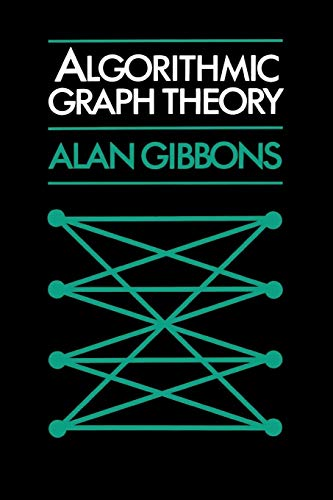Free Shipping in the UK
Proud to be B-Corp

# Algorithmic Graph Theory Alan Gibbons£8.10
New RRP £38.99
Condition - Good
Only 1 left

## Summary

Algorithm Graph Theory introduces most of the classical concepts of pure and applied graph theory (spanning trees, connectivity, genus, colourability, flows in networks, matching and transversals) and covers many of the classical theorems. Its emphasis is on algorithms and their complexity n which graph problems have known efficient solutions and which are intractable.

## Algorithmic Graph Theory Summary

### Algorithmic Graph Theory by Alan Gibbons

This is a textbook on graph theory, especially suitable for computer scientists but also suitable for mathematicians with an interest in computational complexity. Although it introduces most of the classical concepts of pure and applied graph theory (spanning trees, connectivity, genus, colourability, flows in networks, matchings and traversals) and covers many of the major classical theorems, the emphasis is on algorithms and thier complexity: which graph problems have known efficient solutions and which are intractable. For the intractable problems a number of efficient approximation algorithms are included with known performance bounds. Informal use is made of a PASCAL-like programming language to describe the algorithms. A number of exercises and outlines of solutions are included to extend and motivate the material of the text.

## Algorithmic Graph Theory Reviews

'... the book covers a relatively large part of algorithmic graph theory. The theory presented is well motivated. I can conclude that the textbook is well written and for those interested in learning algorithmic graph theory, this is s good introduction.' Zentralblatt fur Mathematik und ihre Grenzgebiete
'... this is a stimulating book for those who want to learn some basic algorithms of graph theory and who already know where to apply them ... nice and thorough introduction.' European Journal of Operational Research
'Alan Gibbons' book ... succeeds admirably.' The Times Higher Education Supplement

Preface; 1. Introducing graphs and algorithmic complexity; 2. Spanning-trees, branchings and connectivity; 3. Planar graphs; 4. Networks and flows; 5. Matchings; 6. Eulerian and Hamiltonian tours; 7. Colouring graphs; 8. Graph problems and intractability; Appendix; Author Index; Subject Index.

GOR006131568
9780521288811
0521288819
Algorithmic Graph Theory by Alan Gibbons
Used - Good
Paperback
Cambridge University Press
19850627
272
N/A
Book picture is for illustrative purposes only, actual binding, cover or edition may vary.
This is a used book - there is no escaping the fact it has been read by someone else and it will show signs of wear and previous use. Overall we expect it to be in good condition, but if you are not entirely satisfied please get in touch with us